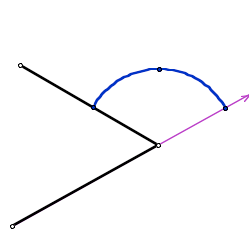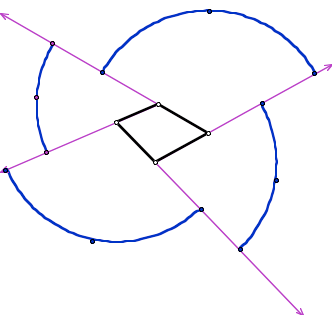SEARCH HOMEMath Central Quandaries & QueriesQuestion from evelina, a parent: how many sides has a regular polygon if the measures of the exterior angle is givenEvelina,

Here is one way to solve the problem. I will include some pictures - because my reasoning is very much visual

In general, for any (convex) polygon, the sum of the exterior angles is 360 degrees
Here is why this is true: Consider walking around the polygon. You turn through the exterior angle at each vertex.When you go all the way around, then you are back facing the same way you started - and have done one complete 'turn'.Now, if the polygon is regular, all the individual corner turns are the same size. With K turns, each turn would would (360/K).

There are alternative ways (with many more steps) which convert through using interior angles - but they have no advantage that I can see!

Walter Whiteley
York UniversityMath Central is supported by the University of Regina and The Pacific Institute for the Mathematical Sciences.